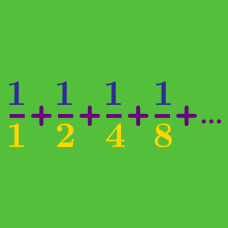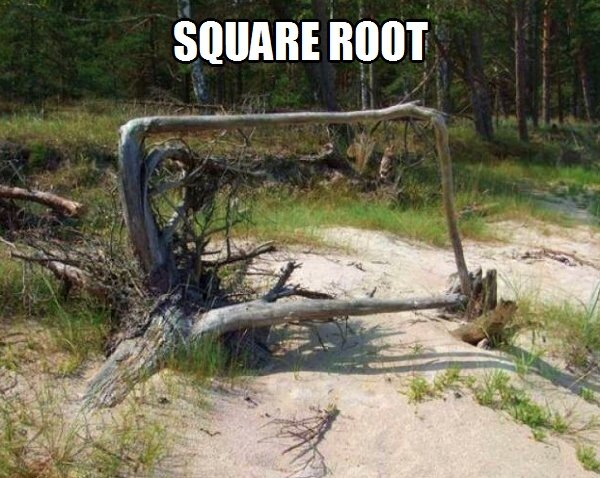Calculus

# Sequences and Series: Level 3 Challenges

If $x^3, x, x^2$ form an arithmetic progression (in the given order), find the sum of all possible values of $x$.

Clarification: An arithmetic progression is allowed to be a constant sequence.

In the answer options, $\phi = \frac{ 1 + \sqrt{5} } { 2}$ (the golden ratio).Cody has started running in a well-organized manner. He runs $100 \text{ m}$ east, then turns left and runs another $10 \text{ m}$ north, turns left and runs $1 \text{ m},$ again turns left and runs $0.1 \text{ m},$ and on the next turn $0.01 \text{ m},$ and so on.

Assuming that Cody can run in this pattern infinitely, the displacement from his initial position can be written as $\frac{a}{\sqrt{b}}$ with $a$ and $b$ being positive integers and $b$ square-free.

What is the value of $a \times b?$

\begin{aligned} 10^{-1}(1 + 0.1 + 0.01& + 0.001 + \cdots) + \\ 10^{-2}(2 + 0.2 + 0.02& + 0.002 + \cdots) + \\ 10^{-3}(3 + 0.3 + 0.03& + 0.003 + \cdots) + \\ &. \\ &. \\ &. \\ \end{aligned}

The above sum can be represented as $\left(\dfrac{a}{b}\right)^{2}$, where $a$ and $b$ are coprime positive integers. Find the value of $a+b$.

Clarification: In the $n^\text{th}$ term, after the string of $n - 1$ zeroes, the number under the bar is $n$.

If the sum of an arithmetic progression of six positive integer terms is 78, what is the greatest possible difference between consecutive terms?$\Large \sqrt{2 \sqrt{4 \sqrt{8 \sqrt{16\ldots}}} } = \, ?$

×